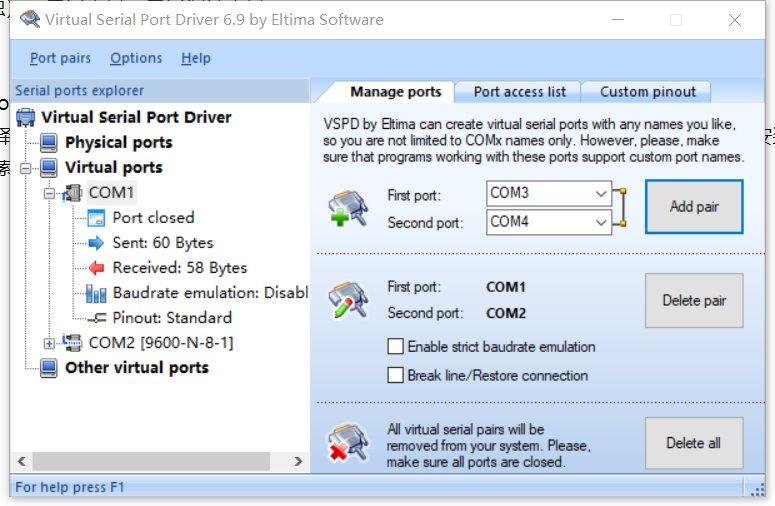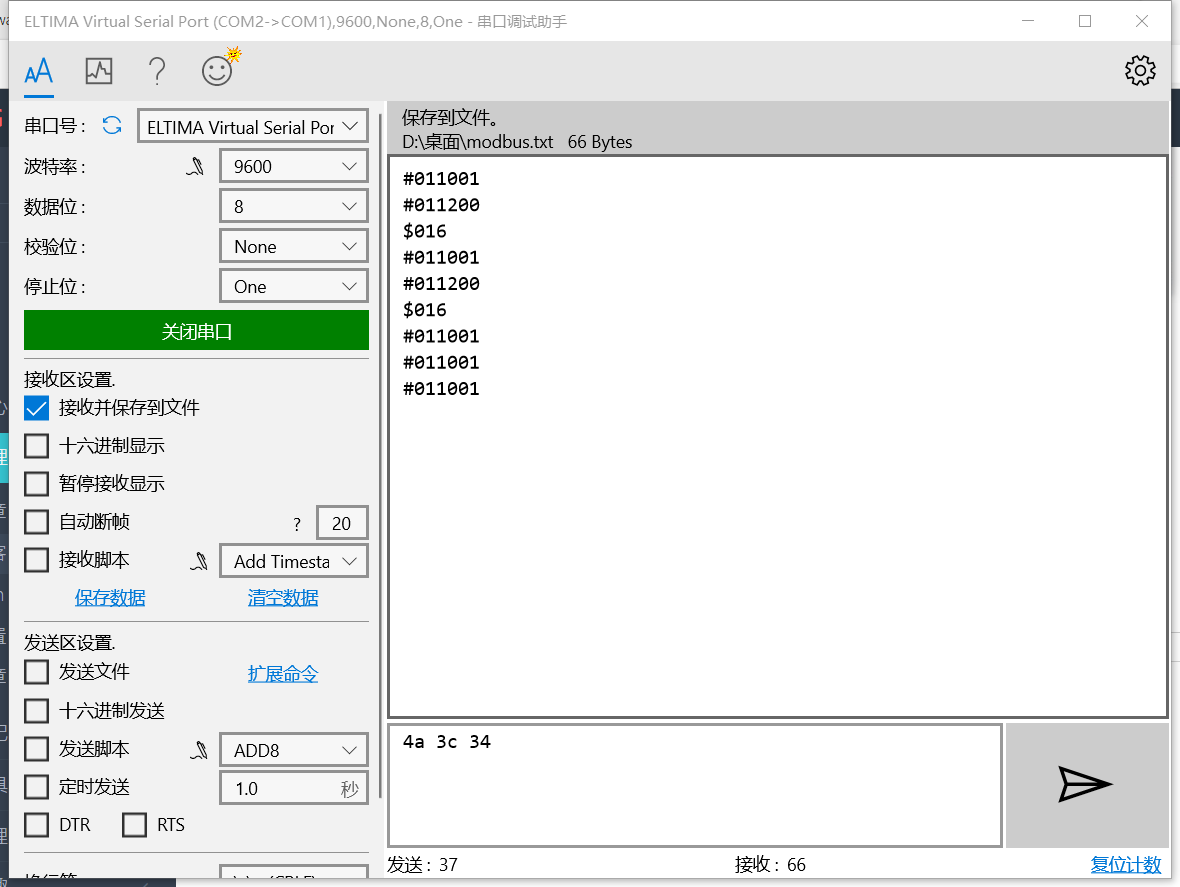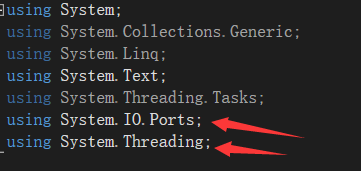×

# 串口通讯

• 串口通讯，又名：串行端口通讯 主要是通过代码控制硬件操作，中间需要进行连接（端口连接）

• 本文主要介绍初步连接端口进行数据交互的操作。即：数据读写

• 所用工具 VS（版本非独），串口工具，串口调试工具

1. 获取虚拟串口工具

• 第一个方法

在电脑上找到：Microsoft Store工具 选择应用 单击搜索串口 选择：超级串口助手  或者 串口调试助手

可以选择购买也可以选择试用，注意：搜索之后没有东西可能是没有登录，工具需要管理员权限，因为涉及到安装

• 第二个方法，在网上搜索串口工具和串口调试工具，资源还是有很多的

• 访问这个网址下载虚拟串口工具

2.配置参数 （参数非独）主要配置参数就是：波特率，数据位，效验位，停止位   数据发送为二进制数据，至于这些参数的意义，可以自行百度
OK,正题来啦 代码到位
3.代码块
创建控制台应用和窗体应用都可以，本例为控制台应用

```using System;
using System.Collections.Generic;
using System.Linq;
using System.Text;
using System.IO.Ports;

namespace ModBus
{
internal class Program
{
static void Main(string[] args)
{
SerialPort serial = new SerialPort();//新建串口对象

string portName = "COM1";//端口号
int baudRate = 9600;//波特率
Parity parity = Parity.None;//校检位
int databits = 8;//数据位
StopBits stopBits = StopBits.One;//终止位
Encoding encodingType = Encoding.ASCII;//编码类型
string SendData;
//------------------------------------------------------
//初始化
serial.PortName = portName;
serial.BaudRate = baudRate;
serial.Parity = parity;
serial.DataBits = databits;
serial.StopBits = stopBits;
serial.Encoding = encodingType;
serial.Open();//打开端口

//发送数据

SendData = "#011001\r"; //设置发送命令 对应DO2通道打开
serial.Write(SendData);//发送命令

//SendData = "#011200\r";//控制DO2通道打开
//serial.Write(SendData);

//SendData = "\$016\r";//查询状态信息（命令）
//serial.Write(SendData);

//接收数据
string content=new UTF8Encoding().GetString(ReDatas);//接收数据转换为字符串
Console.WriteLine(content);

//数据解析
int[] ReDatas_int = new int;

for (int i = 0; i <7; i++)
{
if (content[i]<='9'&&content[i]>='0')
{
ReDatas_int[i] = content[i] - '0';
}
else if (content[i]<='Z'&&content[i]>='A')
{
ReDatas_int[i] = content[i] - 'A' + 10;
}
else
{
ReDatas_int[i]=content[i];
}
}

int[] DOstuas = new int;//定义DO通道状态数组
int wei = ReDatas_int;//取第三位
for (int i = 0; i < 4; i++)
{

DOstuas[i] = wei & 1;
wei= wei>> 1;
}
wei = ReDatas_int;//取第二位
for (int i = 0; i < 4; i++)
{
DOstuas[i + 4] = wei & 1;
wei=wei>> 1;
}
//输出通道状态
for (int i = 0; i < 8; i++)
{
Console.WriteLine("通道DO" + i + ":" + DOstuas[i]);
}

int[] DIstaus = new int;
wei = ReDatas_int;
for (int i = 0; i < 4; i++)
{
DIstaus[i] = wei & 1;
wei = wei>> 1;
}
wei= ReDatas_int;
for (int i = 0; i < 3; i++)
{
DIstaus[i+4]= wei & 1;
wei=(wei>> 1) & 1;
}
//状态输出
for (int i = 0; i < 7; i++)
{
Console.WriteLine("通道DO" + i + ":" + DIstaus[i]);
}

serial.Close();//关闭端口

}
}
}```TNBLOG，技术分享。技术交流：群号677373950
ICP备案 ：渝ICP备18016597号-1